# JEE Main 2020 Question Paper with Solution (Jan 9th first shift)

## 9th January, Morning Session ( 9:30 am to 12:30 pm)

Today is the last day of the JEE main 2020 exam. As we all know the JEE main exam is considering one of the most challenging and competitive exams for engineering courses. The National Test Agency is conducting the JEE Mains 2020 exam in two shifts( Morning and Afternoon) and it is Computer Based Test. The JEE Main 2020 Question Paper with Solution (Jan 9th first shift) are provided by experts on the basis of memory-based JEE main 2020 questions provided by the aspirants. The JEE main 2020 question paper consisted of 75 questions each and 4 marks for each question. All questions were objective type with the single correct option. The Question paper is divided into 3 sections: Chemistry, Physics, and Mathematics. Each section consisted of 25 questions worth 100 marks. Through this article, you will get the 9th January JEE main 2020 question paper with a solution. We suggest you go through the article, so you can check your performance.

Exam date and Shift Article URL

JEE Main 2020 exam - Chemistry ( 9th January first shift)

Q1) The de-Broglie wavelength of an electron in 4th orbit is:

Solution

We have:

n =

n = 4

Q2) The electronic configuration of bivalent Europium and trivalent cerium respectively is:
(Atomic Number : Xe = 54, Ce = 58, Eu = 63)
(1) [Xe]4f7, [Xe]4f1
(2) [Xe]4f7 6s2, [Xe]4f1
(3) [Xe]4f76s2, [Xe]4f15d16s2
(4) [Xe]4f7, [Xe]4f15d16s2

Solution

Eu2+ : [Xe]4f7
Ce3+ : [Xe]4f1

Option 1 is correct.

Q3) Find percentage nitrogen by mass in Histamine?

Solution

The structure of histamine is given below:Molecular formula of Histamine is C5H9N3
Molecular mass of Histamine is 111
Now, mass % of nitrogen = (42/111.15) x 100 = 37.78%

Q4)The major product for above sequence of reaction is:Solution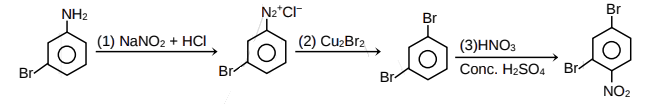Option 2 is correct

Q5) Which of the following will give the highest yield to Friedel Craft's reaction?

(a) C6H5CONH2
(b) Aniline
(c) Chlorobenzene
(d) Phenol

Solution

Haloarenes undergo the usual electrophilic reactions of the benzene ring such as Friedel-Crafts reactions. Halogen atom besides being slightly deactivating is o, p-directing; therefore, further substitution occurs at ortho- and para-positions with respect to the halogen atom.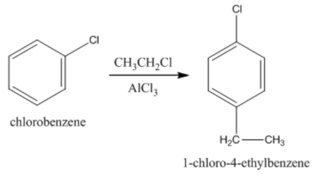Phenol does not undergo Friedel Craft Alkylation or Acylation. This is because oxygen's lone pair in phenol makes coordinate bond with AlCl3 (A Lewis acid) hence blocking it.
Aniline does not undergo Friedel craft's reactions because the reagent AlCl3 (the Lewis acid which is used as a catalyst in friedel crafts reaction), being electron deficient acts as a Lewis base. and attacks on the lone pair of nitrogen present in aniline to form an insoluble complex which precipitates out and the reaction does not proceed.

Therefore, Option(c) is correct.

Q6) For Br2(l)
Enthalpy of atomisation = x kJ/mol
Bond dissociation enthalpy of bromine = y kJ/mole

(a) Does not exist
(b) X>Y
(c) X<Y
(d) X=Y

Solution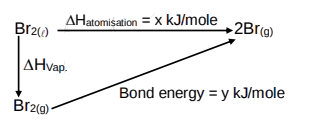Therefore, Option(b) is correct.

Q7) On passing a particular amount of electricity in AgNO3 solution, 108 g of Ag is deposited. What will be the volume of O2(g) in litre liberated at 1 bar, 273k by same quantity of electricity?

Solution

1F charge is required to deposit 1 mole of Ag

Q8) Determine the amount of NaCl to be dissolved in 600g H2O to decrease the freezing point by 0.2°C.
Given : kf of H2O = 2 k-m–1
density of H2O(l) = 1 g/ml

Solution

Q9) Determine degree of hardness in term of ppm of CaCO3 of 10–3 molar MgSO4 (aq).

Solution

10–3 molar MgSO4 = 10–3 moles of MgSO4 present in 1 L solutions.
nCaCO3 = nMgSO4

Q10) Given a solution of HNO3 of density 1.4 g/mL and 63% w/w. Determine molarity of HNO3 solution.

Solution

Q11)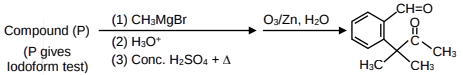Predict the compound (P) on the basis of above sequence of the reactions? Where compound (P) gives positive Iodoform test.Solution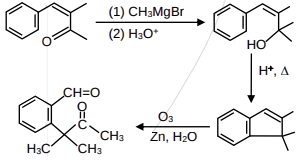Therefore, Option(2) is correct.

Q12) A, B, C and D are four artificial sweetners.
(i) A & D give positive test with ninhydrin.
(ii) C form precipitate with AgNO3 in the lassaigne extract of the sugar.
(iii) B & D give positive test with sodium nitroprusside.

Correct option is:
(1) A – Saccharine, B – Aspartame, C – Sucralose, D – Alitame
(2) A – Aspartame, B – Saccharine, C – Sucralose, D – Alitame
(3) A – Saccharine, B – Aspartame, C – Alitame , D – Sucralose
(4) A – Aspartame, B – Sucralose, C – Saccharine, D – Alitame

Solution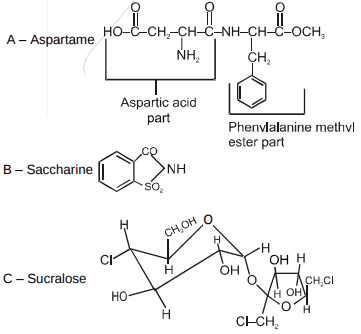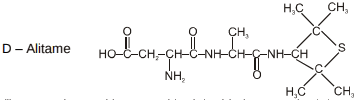(i) A & D give positive test with ninhydrin because both have free carboxylic and amine groups.
(ii) C form precipitate with AgNO3 in the lassaigne extract of the sugar because it has chlorine atoms.
(iii) B & D give positive test with sodium nitroprusside because both have sulphur atoms.

Q13) Write the correct order of basicity: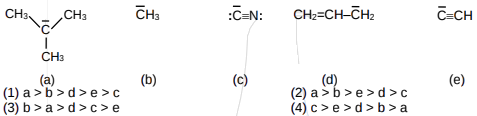Solution

Basicity is inversely proportional to electronegativity.

Therefore, Option(1) is correct.

Q14) Which of the following is the correct order for the heat of combustion?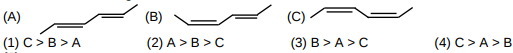Solution
In isomers of hydrocarbon, heat of combustion depends upon their stabilities. As the stability increases heat of combustion decreases.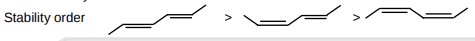Q15)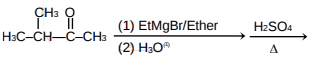What will be the major product?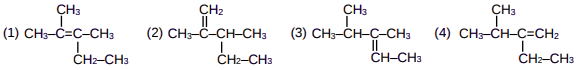Solution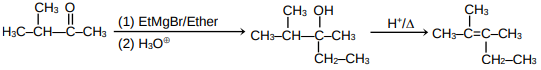Therefore, Option(1) is correct.

Q16. Which of the following species have one unpaired electron each?
(1) O2, O2
(2) O2, O2+
(3) O2+, O2
(4) O2, O22–

Solution

Option 3 is correct.

Q17. Which of the following oxides are acidic, Basic Amphoteric Respectively

(1) MgO, P4O10, Al2O3

(2) N2O3, Li2O, Al2O3

(3) SO3, Al2O3, Na2O

(4) P4O10, Al2O3, MgO

Solution

Non-metal oxides are acidic in nature
alkali metal oxides are basic in nature
Al2O3 is amphoteric.

Option 2 is correct.

Q18. Complex Cr(H2O)6Cln shows geometrical isomerism and also reacts with AgNO3 solution.
Given : Spin only magnetic moment = 3.8 B.M.
What is the IUPAC name of the complex.
(1) Hexaaquachromium(III) chloride
(3) Hexaaquachromium(IV) chloride

Solution

From data of magnetic moment oxidation number of Cr should be +3
Hence complex is Cr(H2O)6Cl3.
Complex shows geometrical isomerism therefore formula of complex is [Cr(H2O)4C2]Cl.2H2O.
It's IUPAC Name: Tetraaquadichloridochromium(III) chloride dihydrate

Option 2 is correct.

Q19.  Ksp of PbCl2 = 1.6 × 10–5

On mixing 300 mL, 0.134M Pb(NO3)2(aq.) + 100 mL, 0.4 M NaCl(aq.)

(1) Q > Ksp

(2) Q < Ksp

(3) Q = Ksp

(4) Relation does not exit

Solution

So, Q > Ksp

Option 1 is correct

Q20. Which of the following can not act as both oxidising and reducing agent ?
(1) H2SO3

(2) HNO2

(3) H3PO4

(4) H2O2

Solution

As in H3PO4 Phosphorous is present it's maximum oxidation number state hence it cannot act as reducing agent.

Option 3 is correct.

Q21.  First Ionisation energy of Be is higher than that of Boron.
Select the correct statements regarding this
(i) It is easier to extract electron from 2p orbital than 2s orbital
(ii) Penetration power of 2s orbital is greater than 2p orbital
(iii) Shielding of 2p electron by 2s electron
(iv) Radius of Boron atom is larger than that of Be

(1) (i), (ii), (iii), (iv)

(2) (i), (iii), (iv)

(3) (ii), (iii), (iv)

(4) (i), (ii), (iii)

Solution

Concept based

Option 4 is correct.

Q22. [PdFClBrI]2– Number of Geometrical Isomers = n. For [Fe(CN)6]n–6 , Determine the spin only magnetic moment and CFSE (Ignore the pairing energy)

Solution

Number of Geometrical Isomers in square planar [PdFClBrI]2– are = 3
Hence, n = 3
[Fe(CN)6]3–
Fe3+ = 3d5, According to CFT configuration is

Option 1 is correct.

Q23. A can reduce BO2 under which conditions.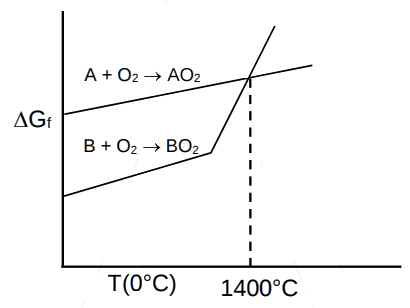(1) > 1400°C

(2) < 1400°C

(3) > 1200°C and < 1400°C

(4) < 1200°C

Solution

Option 1 is correct.

Q24.

Rate of reaction in absence of catalyst at 700 K is same as in presence of catalyst at 500 K. If catalyst decreases activation energy barrier by 30 kJ/mole, determine activation energy in presence of catalyst.

(Assume 'A' factor to be same in both cases)

(1) 75 kJ

(2) 135 kJ

(3) 105 kJ

(4) 125 kJ

Solution

Activation energy of the catalysed reaction = 105 – 30 = 75 kJ/mole

Option 1 is correct

Q25. A substance 'X' having low melting point, does not conduct electricity in both solid and liquid state. 'X' can be:

(1) Hg

(2) ZnS

(3) SiC

(4) CCl4

Solution

Option 4 is correct

JEE Main 2020 exam - Mathematics ( 9th January first shift)

Q1. The number of 5 digit numbers can be formed using distinct digits is 336K. Value of K is such that 2 occupies tens place

Solution:

1st term can be(1,3,4.....9) and 2nd can be (0,1,3...9 but one digit used to fill 1st term) and tens place can be filled by only a single digit 2

336K=8X8X7X6X1
so k=8

Q2.

Solution:

Q3. A spherical iron ball 10 cm in radius is coated with a layer of ice of uniform thickness than melts at a
rate of 50 cm3/min. When the thickness of ice is 5 cm, then the rate at which the thickness of ice decreases is

Solution:

Q4. The coefficient of  in the expansion of   is ?

Solution:

Q5. The distinct solution of the equation  ?

Solution:

Total number of solution is 8

Q6. A card is picked at random with replacement till the second A card appears.Find the probability that the second A card appears before 3rd B card

Solution:

Possible outcomes are: AA + ABA + BAA + ABBA + BBAA + BABA

Q7. Value of

Solution:

Q8.

Then the value of k is

Solution:

k=16

Q9.

Solution:

Q10.

Solution: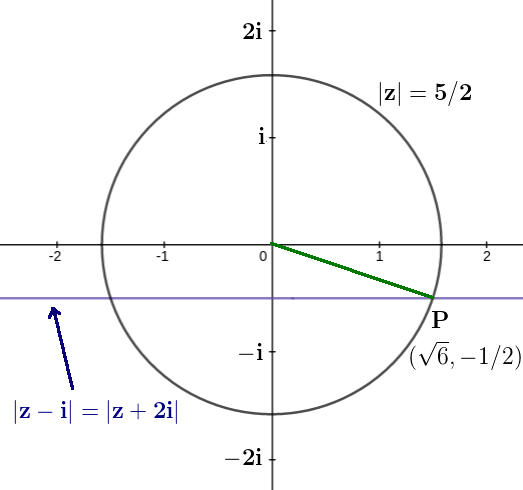z =

Q11. Value of is

Solution:

Q12. If f(x) = a + bx + cx2 where a, b, c  then  is

Solution:

f(1) = a + b + c

f(0) = a

Option (2) satisfies the given condition

Q13. , If y(2)=0 then y(3)=?

Solution:

So at x=3 y=3

Q14.

Solution:

Q15. If the plane  intersects in a line (R × R × R) then  is equal to

Solution:

Q16. For observations xi given  If mean and variance of observations (x1 –3), (x2 –3) ….…..(x10 –3) is  respectively then ordered pair is

Solution:

Q17. A (3,–1), B(1,3), C(2,4) are vertices of ABC if D is centroid of ABC and P is point of intersection of lines x + 3y – 1 = 0 and 3x – y + 1 = 0 then which of the following points lies on line joining D and P

(1) (–9,–7)

(2) (–9,–6)

(3) (9,6)

(4) (9,–6)

Solution:

The centroid of triangle ABC D(2,2)

Point of intersection P

equation of line DP is  8x – 11y + 6 = 0

Point  (–9,–6) satisfies the equation

Q18. If f(x) is twice differentiable and continuous function in x [a, b] also f'(x) > 0 and f ''(x) < 0 and c  [a, b] then  is greater than

Solution:

Use LMVT for x [a,c]

also use LMVT for x [c,b]

f ''(x) < 0 f '(x) is decreasing

Q19. If points A (2, 4, 0), B(3, 1, 8), C(3, 1, –3), D(7, –3, 4) are four points then the projection of line segment AB on line CD.

Solution:

Q20. If and are coplanar vectors and  then value of  is ?

Solution:

are coplanar vectors so

By solving this a=-1/3 so now

By solving you will get

=1

Q21.  and C=3A then  is?

Solution:

Q22. Find number of real roots of equation  is?

Solution:

Let

Now the equation

Let

Only positive value possible so

x=0 is the only solution.

Q23. The negation of ‘ is an integer or is an irrational number’ is

(1) is an integer and is not an irrational Number

(2) is not an integer and is an irrational Number

(3) is not an integer or is not an irrational Number

(4) is not an integer and is not an irrational Number

Solution:

is not an integer and 5 is not an irrational Number

or

Q24. If  and f(0) = 0 then the value of f(1) is

Solution:

Q25. If a circle touches y-axis at (0, 4) and passes through (2, 0) then which of the following can not be the tangent to the circle

(1) 3x + 4y – 6 = 0                (2) 3x + 4y – 24 = 0

(3) 4x – 3y – 17 = 0              (4) 4x + 3y – 6 = 0

Solution: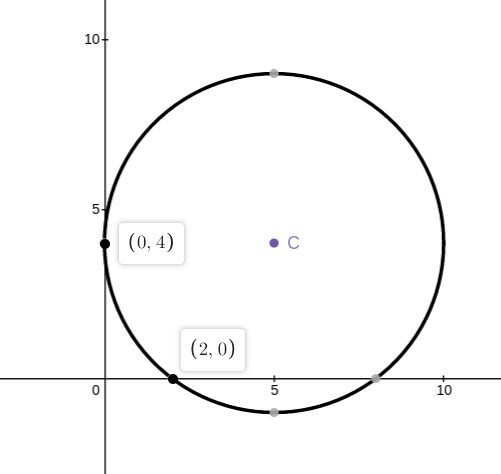Equation of family of circle touches at (0,4)

This family of circle passes through (2,0)

Now check the option

Option 1 is the correct option

JEE Main 2020 exam - Physics ( 9th January first shift)

Q-1

(1) 1.8 eV (2) 0.8 eV (3) 1.1 eV (4) 1.4 eV

Solution-

From photoelectric equation:-

And

Using

and radius in terms of V can be written as :-

So

So W=1.1 eV

Q-2

Solution:-

Q-3

The kinetic energy of the particle is E and it's De-Broglie wavelength is . On increasing it's KE by , it's new De-
Broglie wavelength becomes    . Then   is

1) 3E   2) E  3) 2E 4) 4E

Solution-

Q-4

Solution-

Q-5

Solution-

Q-6

Three identical solid spheres each having mass 'm' and diameter 'd' are touching each other as shown
in figure. Calculate ratio of moment of inertia about an axis (perpendicular to plane of paper) passing
through point P and B as shown in fiven P is centroid of triangle ABC.Solution

As a moment of inertia of one sphere of radius R about its centre=

from the figure given it is clear that

Q-7

Consider an infinitely long current carrying cylindrical straight wire having radius 'a'.Then the ratio of

magnetic field at distance    and 2 a from axis of wire is.

Solution-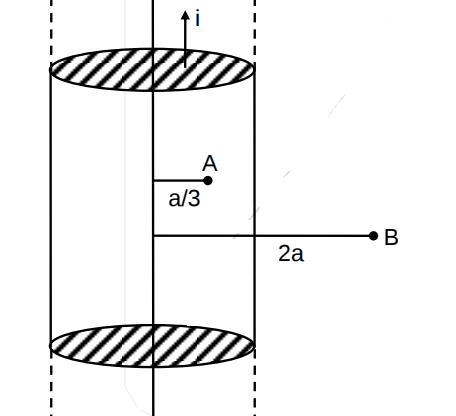Q-8

Find current in the wire BC.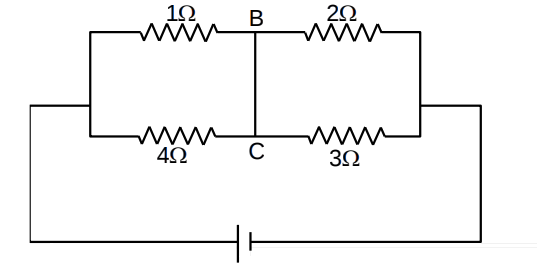(1) 1.6A (2) 2A (3) 2.4A (4) 3A

Solution-

So after drawing the circuitQ-9

Two electromagnetic waves are moving in free space whose electric field vectors are given by

A charge q is moving with velocity  Find the

net Lorentz force on this charge at   and when it is at origin.

Solution-

Magnetic field vectors associated with this electromagnetic wave are given by

Q-10]

Solution:-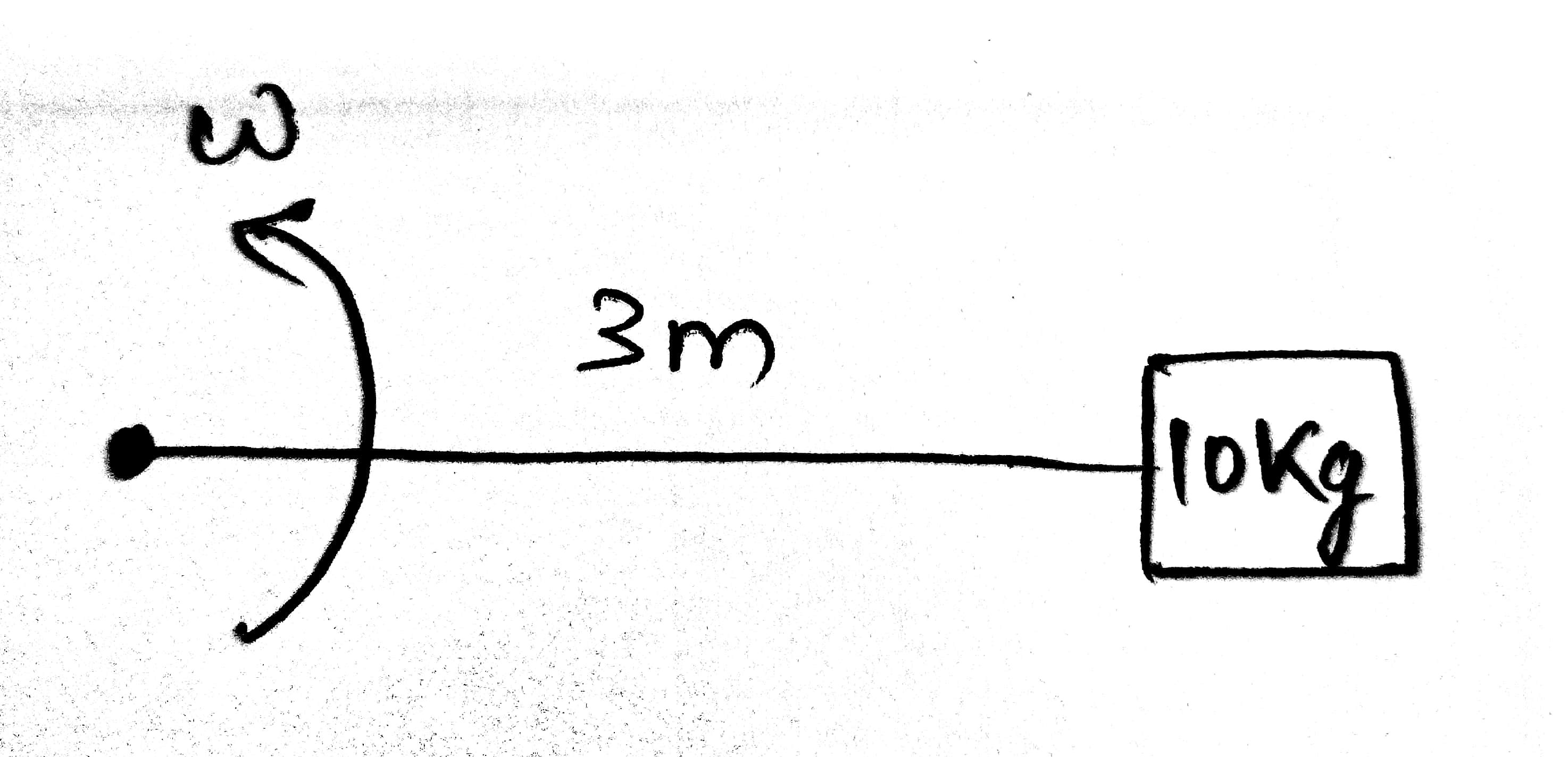Q-11

A sphere of uniform charge density   and radius R has a cavity of radius R/2 as shown in figure. Find the ratio of magnitude of electric field at A and B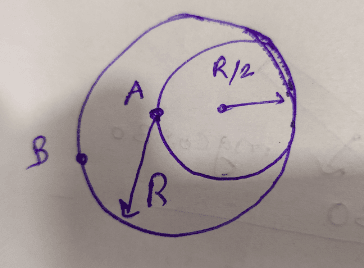Solution:-

Condider a sphere of density  and radius R/2 is removed from a sphere of radius R

then

Electric field at A is

Q-12

Two ideal di-atomic gases A and B. A is rigid, B has an extra degree of freedom due to vibration. Mass of A is m and mass of B is 4m . The ratio of molar specific heat of A to B at constant volume is :

Solution -

Q-13

An ideal liquid (water) flowing through a tube of non-uniform cross section area at A and B are 40 cm2 and 20 cm2 respectively. If pressure difference between A & B is 700 N/m2 then volume flow rate is :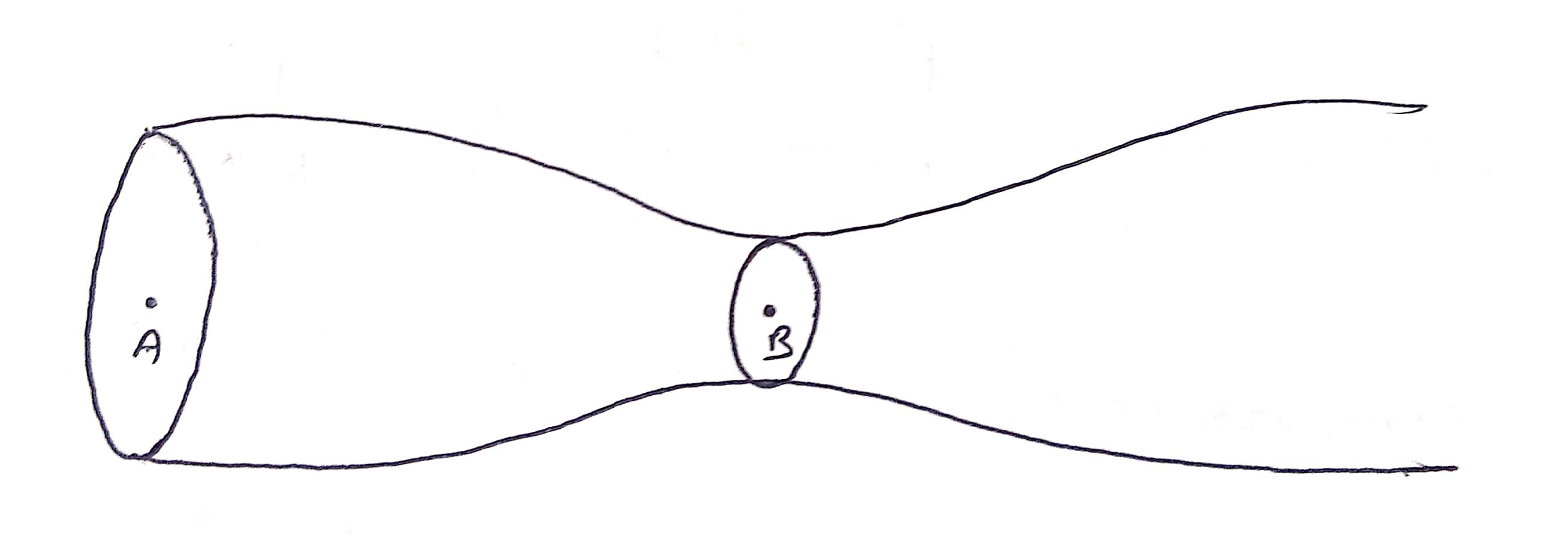Solution -

Q-14

A telescope of aperture diameter 5m is used to observe the moon from the earth. Distance between the moon and earth is 4 × 105 km. Determine the minimum distance between two points on the moon's surface which can be resolved using this telescope. (Wave length of light is 5893 Å.)

Solution -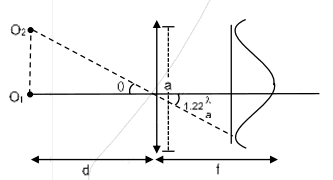Option (1) is correct.

Q-15

A particle of mass m is revolving around a planet in a circular orbit of radius R. At the instant the particle has velocity  , another particle of mass  moving at velocity  collides perfectly in-elastically with the first particle. The new path of the combined body will take is

(1) Circular                        (2) Elliptical                       (3) Straight line                        (4) Fall directly below on the ground

Solution -

Option (2) is correct

Q-16

Two particles of same mass 'm' moving with velocities  and   collide in-elastically. Find the loss in kinetic energy.

Solution -

So option (1) is correct.

Q-17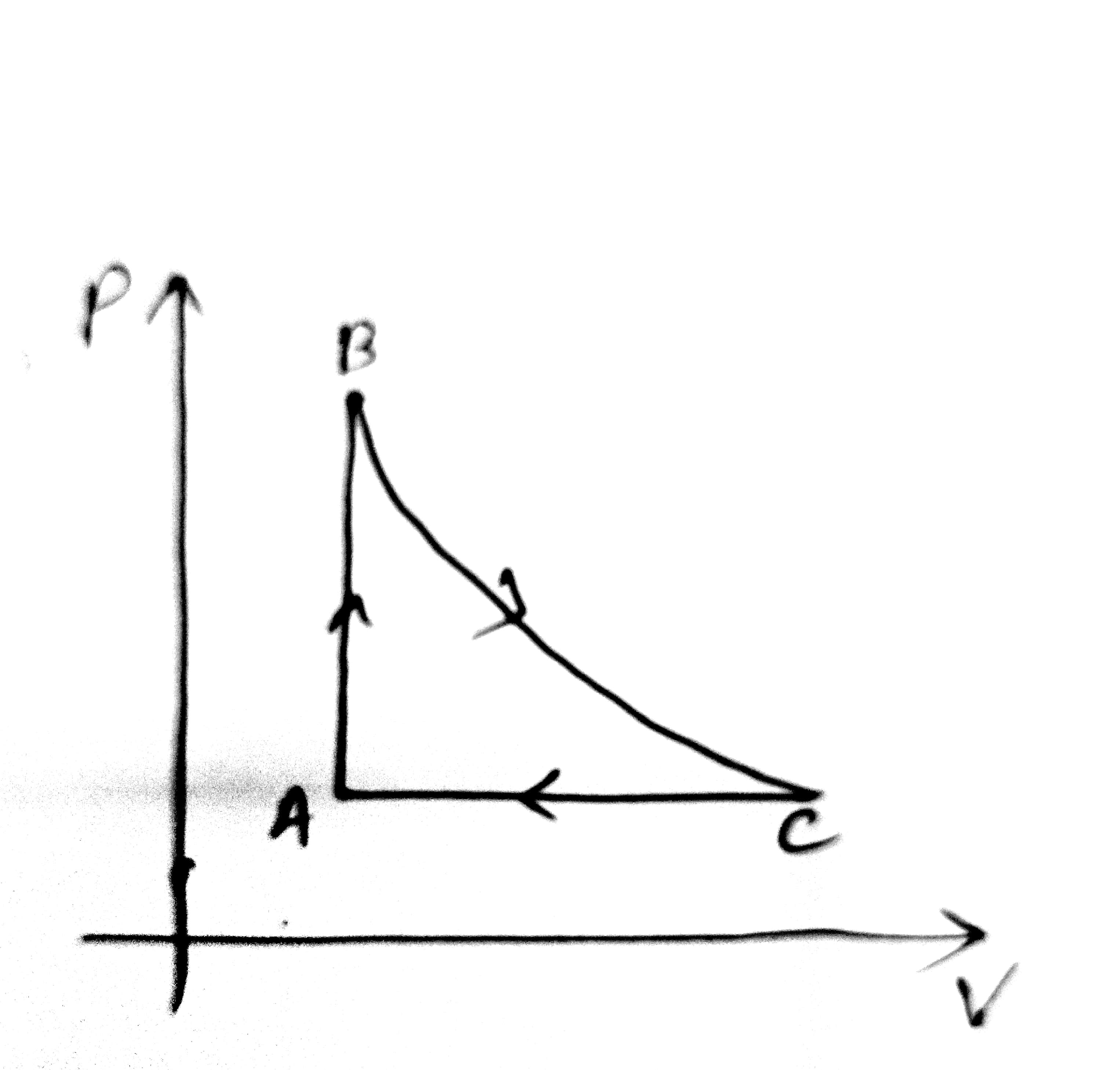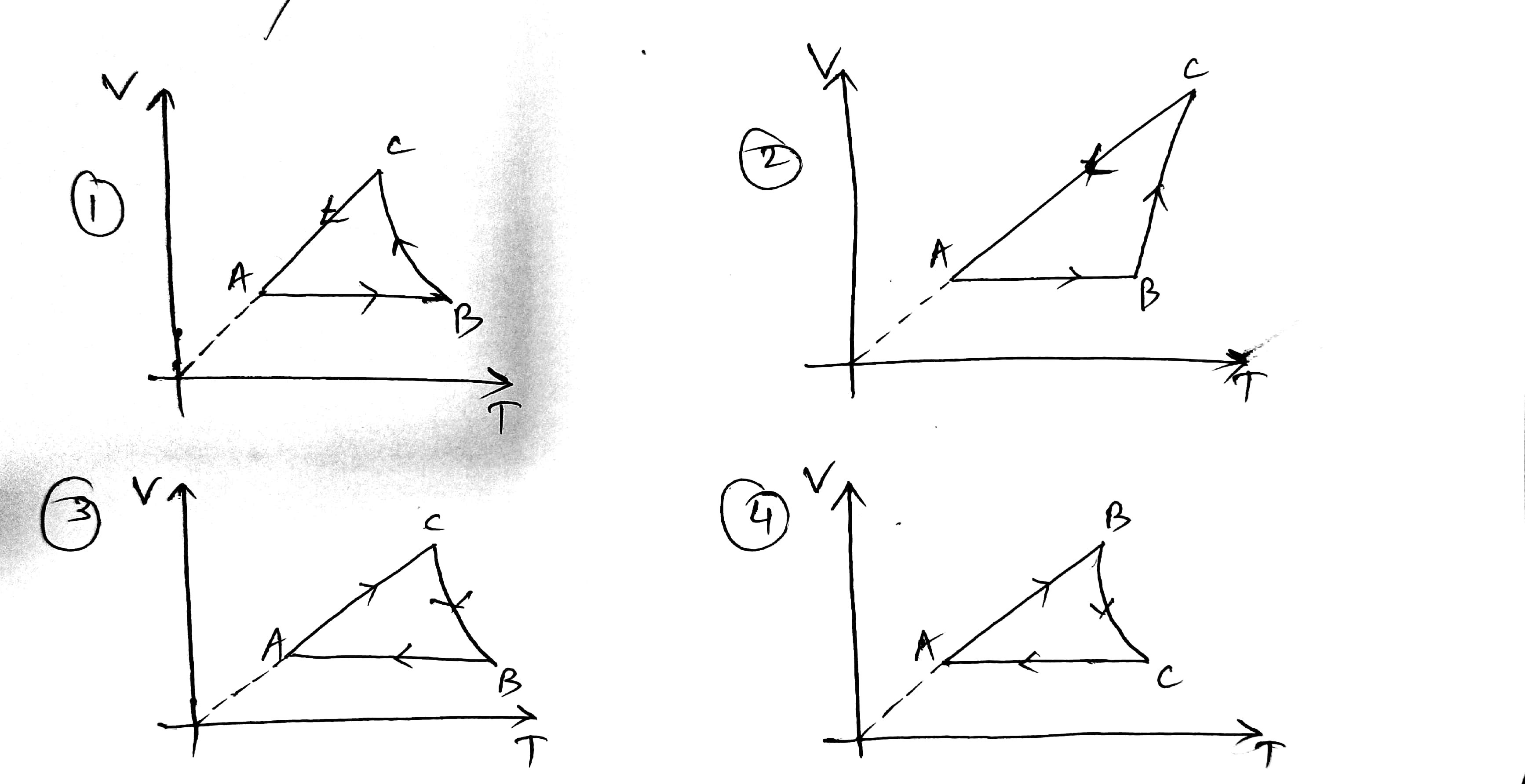Solution:-

So, option 1 is correct.

Q-18

Three waves of same intensity (I0) having initial phases  rad respectively interfere at a point. Find the resultant Intensity.

options:

(1) I0 (2) 0 (3) 5.8 I0 (4) 0.2 I0

Solution-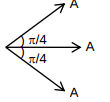Q-19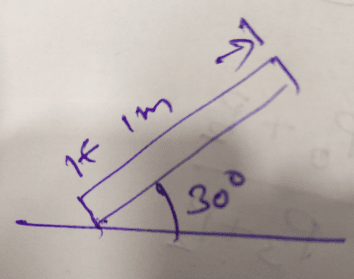Solution:-

Q-20

If reversible voltage of 200 V is applied across an inductor, current in it reduces from 0.25A to 0A in 0.025ms. Find inductance of inductor (in mH).

Solution:-

Q-21

Position of a particle as a function of time is given as x2 = at2 + 2bt + c, where a, b, c are constants. Acceleration of particle varies with x–n then value of n is.

Sol:

Again differentiating with respect to t

Q-22

Given  and  . Find vector parallel to electric field at position [Note that   ]

sol:

E must be antiparallel to p

Q-23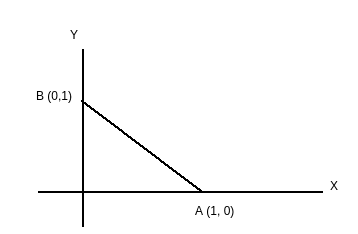Solution:-

Q-24

In the given circuit both diodes are ideal having zero forward resistance and built-in potential of 0.7 V. Find the potential of point E in volts.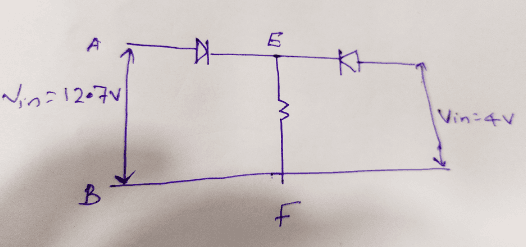sol-

By applying kvl in ACBFA12.7-0.7-VEF=0.

implies

VEF=12V

Q-25

Which of the following statements are correct for moving charge as shown in figure.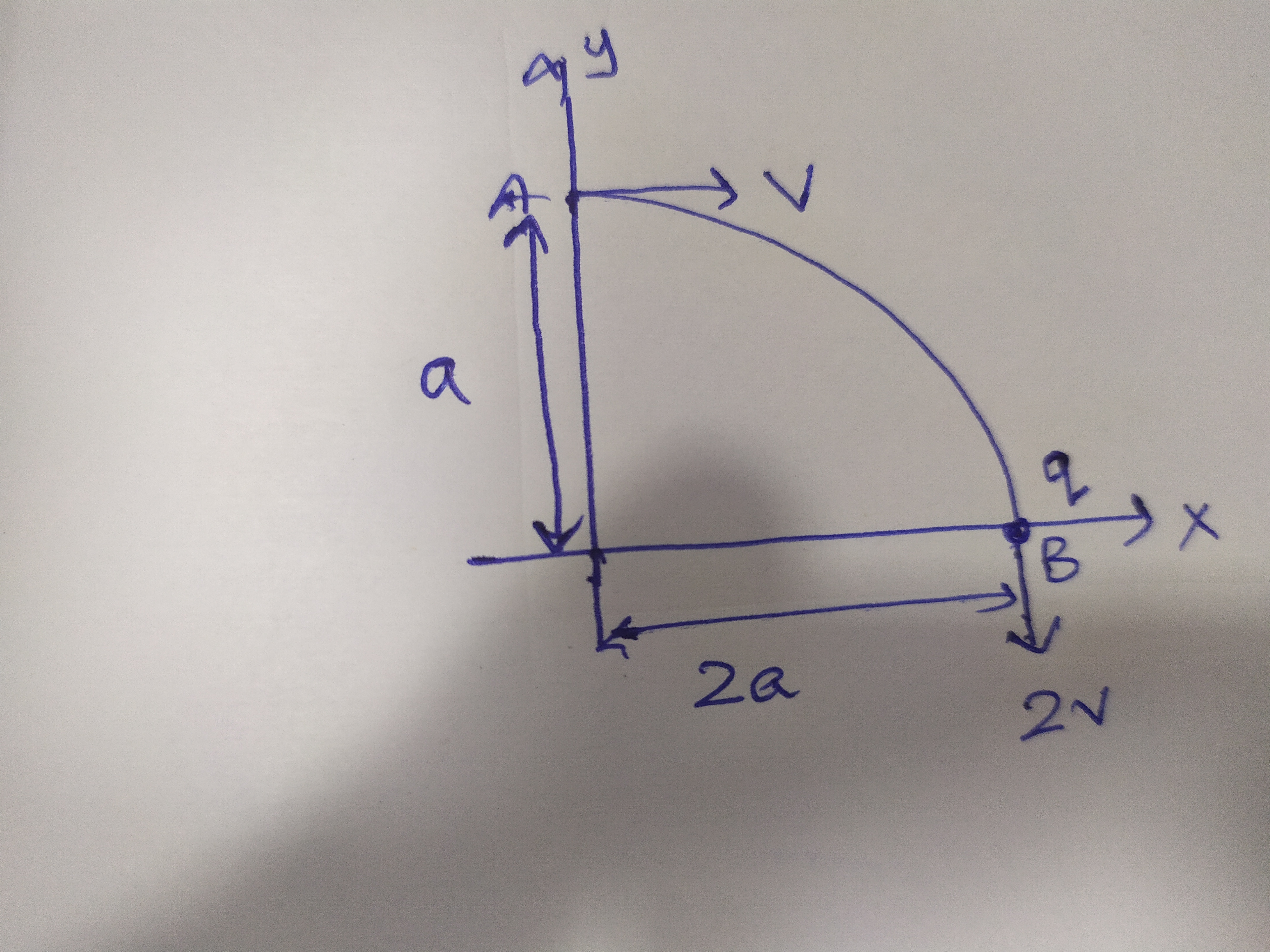(1) A, B, C are correct (2) A, B, C, D are correct (3) A, B are correct (4) B, C, D are correct.

Solution-

A) by work energy theorem

So option (1) is correct.

Stay tuned with us for further updates.